|

# 地暖专用 大卫纯实木地暖地板——番龙眼评测

前几天上海气温最高达到40.9℃，打破了上海有气象记录以来最高温的记录。炎炎夏日，大概谁都不会想起寒冷的感觉。可是你知道吗？专家说今年的冬天，可能会把你冻哭哦！研究显示，拉尼娜将使得我国大部出现冷冬热夏，这个寒冷的冬天，我们该如何度过呢？一直以来，空调暖风干燥、耗电高被人所诟病，现在越来越多的人开始选择地暖。地热取暖更加科学合理，舒适健康，经济节能。当然，想要享受地暖带来的温暖，首先需要有优质的、适合地暖的地板。今天搜狐焦点家居将评测一款这样的地板，环保兼稳定，一起来看看吧。【产品信息】

产品名称：大卫纯实木地暖地板——番龙眼 珊瑚海洋

系列型号：S29G41-F7

规格参数：910x115x18

木材来源：东南亚气干密度：0.6-0.74g/cm3

【外观细节】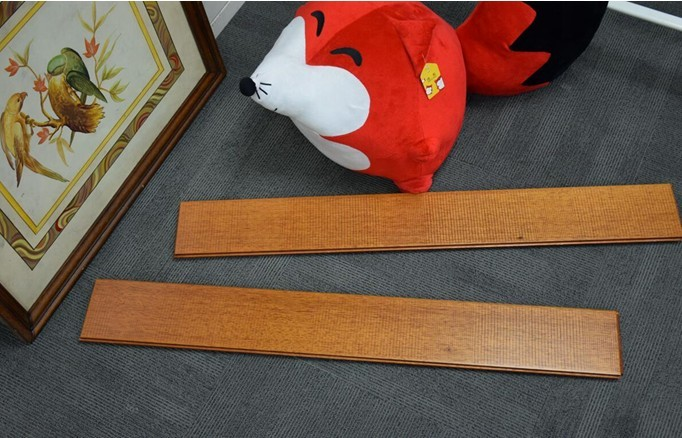观察地板外观，呈黄褐色，漆面通透清晰，细腻饱满，光泽度温润柔和。番龙眼不仅具有东方气质，还有一个具有东方特色的俗称：唐木。从其俗称上，就可以看出其中式典雅的风格。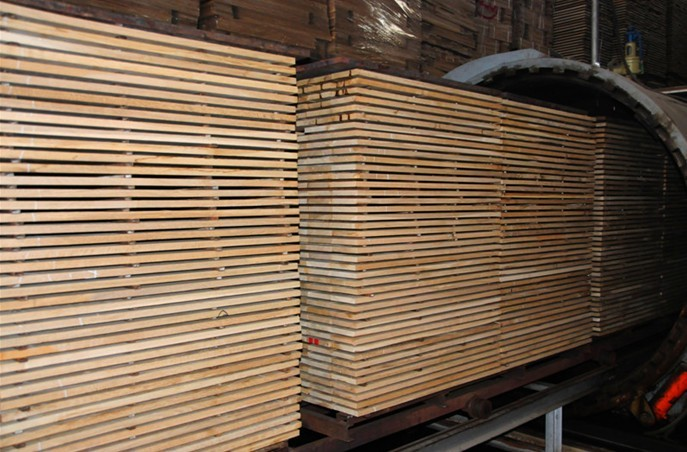为配合地板在地暖情况下使用，地板经过了微波热处理技术，在隔绝氧气的条件下将木材加热到130℃-160℃左右进行处理，科学保持实木地板天然木纤维,合理控制原木含水率，与碳化木相比，既保留了木头原有的“活性”，又大大增加了木头的稳定性。不易起翘、不易变形。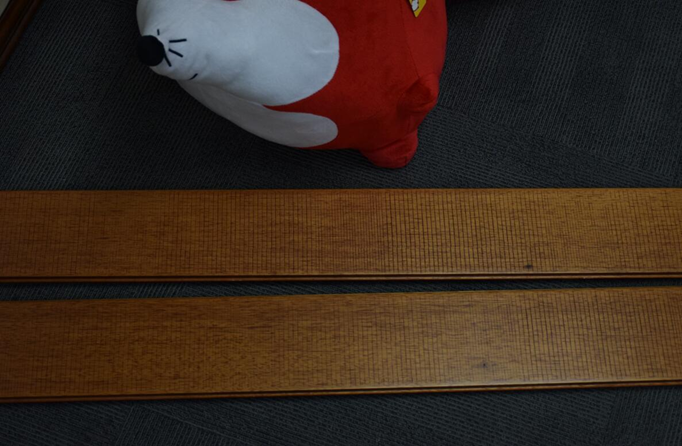地板纹理美观，错落有致，整体搭配视觉效果出色。表面锯齿状仿古工艺，仿佛饱经沧桑的历练，让地板更有触感，更贴近自然的木材本身。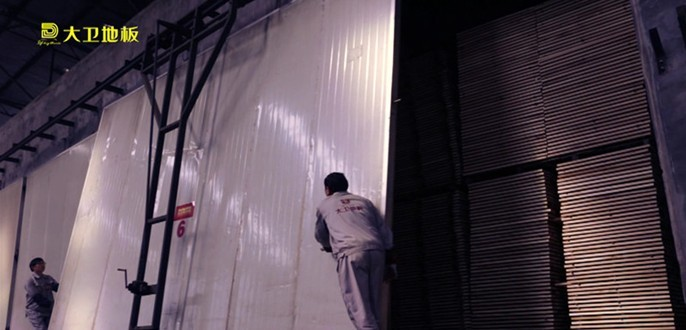地板还经过“微波烘干”窑，在温度35℃-40℃，相对湿度90%的平衡窑程序中处理至少25天，让其释放产品内部的剩余的应力，使其在地暖情况下更稳定。养生平衡是所有工序里最为重要的环节，是深入产品质量的真功夫。抚摸地板表面，手感温润，纹理虚实相间，让其内敛的美感油然而生，处处散发着东方气质，宛若一位优雅的东方佳人，极具品格高雅之韵。试想一下，冬季在温暖的房间里，赤脚踩在装有地暖的地板上，温润的脚感，应该十分美妙。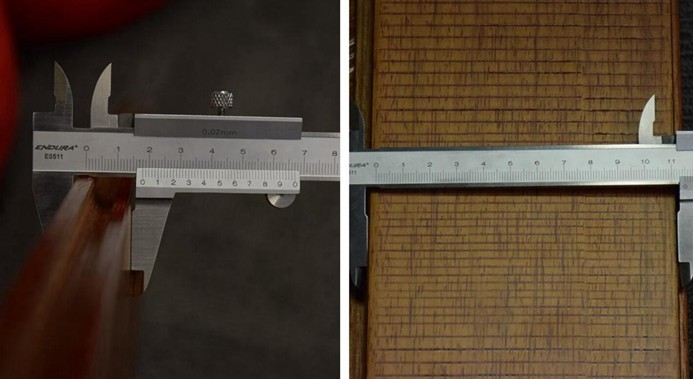地板的厚度，直接关系到地板的用材，用尺测量出一块地板的长为910mm，宽为115mm，厚度约为18mm，符合产品所标注尺寸，非常厚实。作为国内最知名的地板品牌之一，大卫地板的技术实力毋庸置疑，先进的设备和雄厚的技术实力也是其产品品质最有力的保障。大卫地板引进德国八轴威力四面刨，并与德国蓝帜刀具签订战略合作，先进的微精度切割工艺，产品精度以丝米计量，确保品质出众。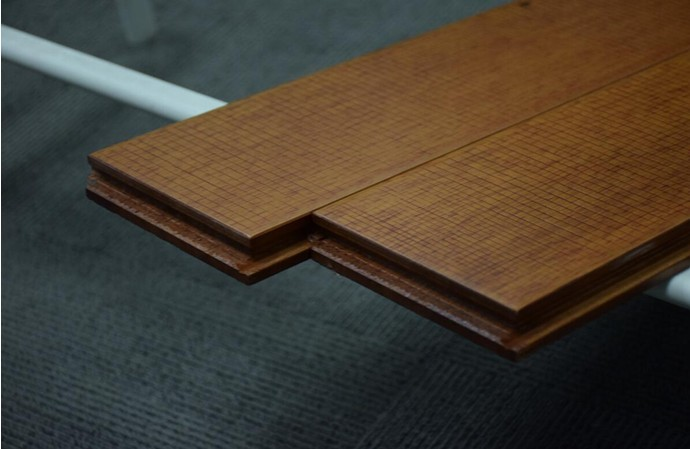由于热胀冷缩的变动，地暖地板需要比一般地板锁扣更加牢固。这款地板锁扣经过特别设计，锁扣更大，双层保证，一扣既合，将两片地板板牢牢扣住，更紧密，不易拔缝、不易起翘，充分适应地暖环境。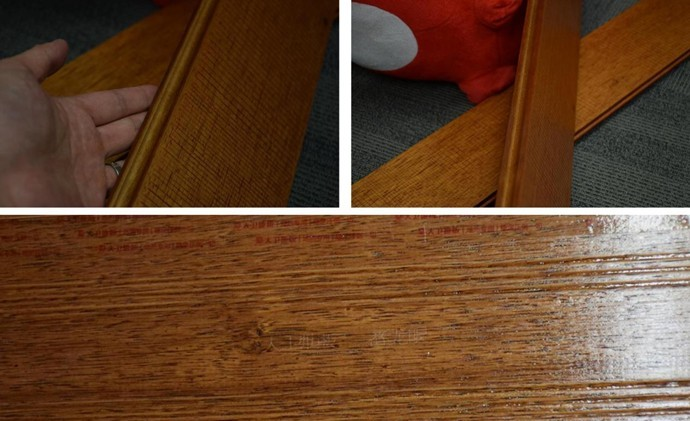观察地板的各个边角处十分规整，无毛刺，纯实木材质。地板背面有大卫地板番龙眼的防伪字样。大卫地板采用树种实名制，严格按照国家要求的树种规范名称及等级，将树种名称通过激光刻印于背板上，让消费者在购买时可以更加清楚地板的木种，保证品质。【使用性能】评测完了大卫纯实木地暖地板——番龙眼的外观和细节，接下来搜狐焦点家居将对该地板的耐磨、抗污、防水和防火阻燃等方面的性能进行测试，来看下这款地板在基本性能方面的具体表现吧。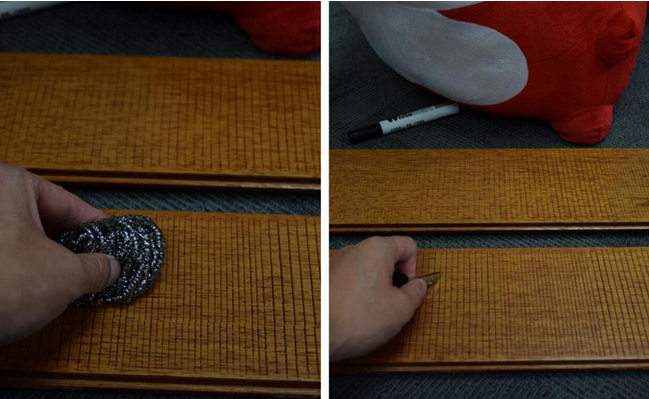耐磨度测试

分别使用常见的钥匙和清洁球，使用其尖锐的顶部对着地板表面用力划擦30秒，地板表面未出现明显的划痕，也没有漆膜碎屑产生，可见这款强化地板的耐磨性能不错。大卫地板使用德国坚弗环保油漆，辅以大卫油漆工艺，确保大卫地板表层油漆更饱满，纹理清晰度更高，又提高了地板的耐磨性、耐撞性和柔韧性。抗污力测试

接下来，我们通过笔迹擦除情况来测试地板的抗污能力。首先我们在这款地板上写上“大卫”字样。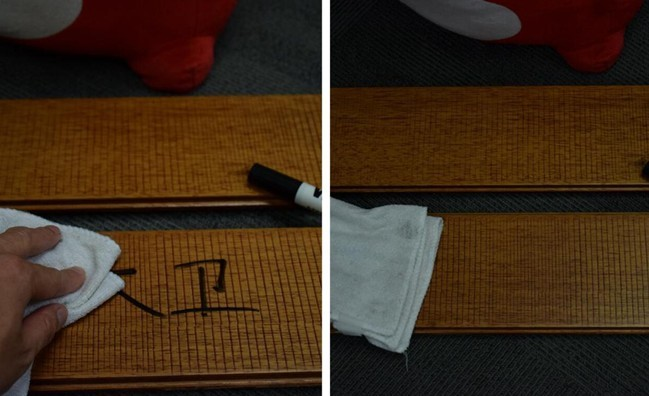静置几分钟待其略干，使用抹布轻轻擦拭，字迹便可以完全清除。可见这款地板的抗污力不错。我们也可以看到擦拭后抹布上的黑色墨迹。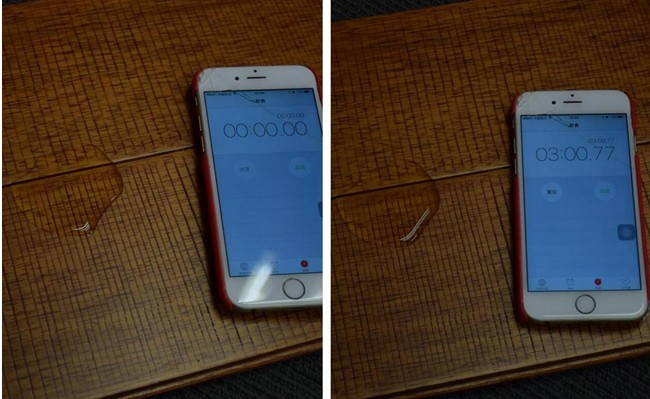防水性测试

滴一滴清水在两块地板的拼接处，表面静置几分钟，观察水珠并无明显滚动和渗透的现象，擦拭干净之后地板表面未出现变色情况，此款地方的防水性不错。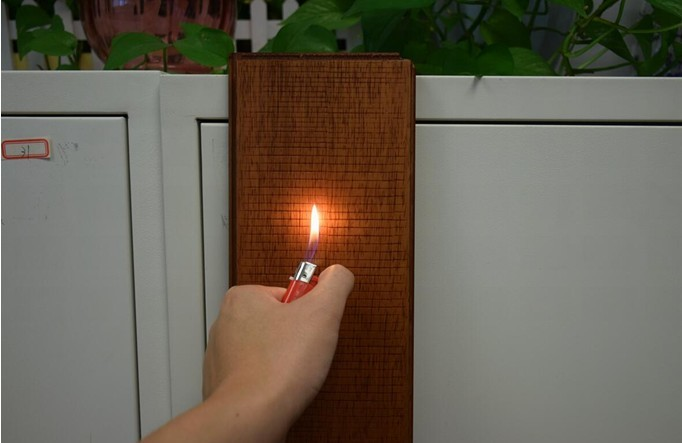防火阻燃测试

将打火机开至大火对着地板表面灼烧，观察表面是否有燃烧和起泡现象，打火机灼烧三秒，地板表面未出现起泡现象和黑焦情况。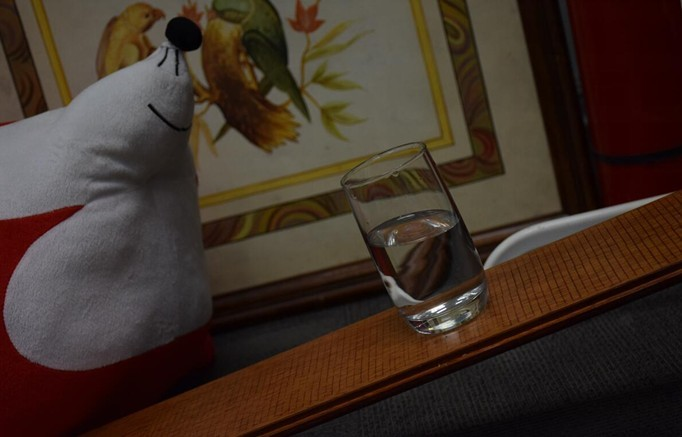防滑测试

将地板约30度角倾斜放置，然后将装有半杯水的水杯放在地板表面，观察玻璃杯仍未出现滑落的迹象，可见此款地板的防滑性不错。防潮更出色，抗刮更清晰。大卫纯实木地暖地板采用德国坚弗环保漆，行业顶级的160米长意大利进口现代化淋漆线，能够一次性满足工艺要求的14道程序，正面“9底5面”，背面“2底2面”，四边封漆，360度保护，让地板更好的抵挡潮气入侵，提升品质，使用更长久。

【评测总结】岁月珍稀原木，把森林搬回家。大卫纯实木地暖地板——番龙眼采用东南亚名贵木材番龙眼，以纯实木打造，外观典雅柔和，极具东方特色。地板经过微波热处理、微波烘干技术处理；地板导热双向均匀，热传导更快，足下升温，自下而上，调节温度，配合大锁扣的稳定性，使其具有适合地热的独特优势。在基本性能方面，地板在耐磨、抗污、防水和防火阻燃等各方面测试中都有不俗的表现。这个冷冬快到了！感兴趣的朋友可以了解和选购哦。【品牌简介】

大卫地板，致力于“创造精致家居”，让每个中国人享受精致家居生活。年产能达1000万平方米，产品涵盖纯实木地热、实木、多层、仿古、强化、安装材料、地板保养产品等七大品类60多个系列1000多种产品。地板行业是良心产业，做地板就是做良心！大卫是业内为数不多的几家公司检测中心通过国家CNAS实验室认证的企业，实验结果可获全球54个国家与地区实验室认可，品质保证更权威！专注地板19年，是《实木地板通用要求》国际标准、《地采暖用实木地板技术要求及铺装使用规范》等4项国家标准以及5项行业标准主要起草单位，大卫地板在创新中不断超越，以环保、精致为内涵，将纯实木地暖、F4星、E0级产品带给全世界，有品质更健康，为无数家庭带来满心的幸福与愉悦。

`声明：本文由入驻焦点开放平台的作者撰写，除焦点官方账号外，观点仅代表作者本人，不代表焦点立场错误信息举报电话： 400-099-0099，邮箱：jubao@vip.sohu.com，或点此进行意见反馈，或点此进行举报投诉。`A B C D E F G H J K L M N P Q R S T W X Y Z
A - B - C - D - E
• A
• 鞍山
• 安庆
• 安阳
• 安顺
• 安康
• 澳门
• B
• 北京
• 保定
• 包头
• 巴彦淖尔
• 本溪
• 蚌埠
• 亳州
• 滨州
• 北海
• 百色
• 巴中
• 毕节
• 保山
• 宝鸡
• 白银
• 巴州
• C
• 承德
• 沧州
• 长治
• 赤峰
• 朝阳
• 长春
• 常州
• 滁州
• 池州
• 长沙
• 常德
• 郴州
• 潮州
• 崇左
• 重庆
• 成都
• 楚雄
• 昌都
• 慈溪
• 常熟
• D
• 大同
• 大连
• 丹东
• 大庆
• 东营
• 德州
• 东莞
• 德阳
• 达州
• 大理
• 德宏
• 定西
• 儋州
• 东平
• E
• 鄂尔多斯
• 鄂州
• 恩施
F - G - H - I - J
• F
• 抚顺
• 阜新
• 阜阳
• 福州
• 抚州
• 佛山
• 防城港
• G
• 赣州
• 广州
• 桂林
• 贵港
• 广元
• 广安
• 贵阳
• 固原
• H
• 邯郸
• 衡水
• 呼和浩特
• 呼伦贝尔
• 葫芦岛
• 哈尔滨
• 黑河
• 淮安
• 杭州
• 湖州
• 合肥
• 淮南
• 淮北
• 黄山
• 菏泽
• 鹤壁
• 黄石
• 黄冈
• 衡阳
• 怀化
• 惠州
• 河源
• 贺州
• 河池
• 海口
• 红河
• 汉中
• 海东
• I
• J
• 晋中
• 锦州
• 吉林
• 鸡西
• 佳木斯
• 嘉兴
• 金华
• 景德镇
• 九江
• 吉安
• 济南
• 济宁
• 焦作
• 荆门
• 荆州
• 江门
• 揭阳
• 金昌
• 酒泉
• 嘉峪关
K - L - M - N - P
• K
• 开封
• 昆明
• 昆山
• L
• 廊坊
• 临汾
• 辽阳
• 连云港
• 丽水
• 六安
• 龙岩
• 莱芜
• 临沂
• 聊城
• 洛阳
• 漯河
• 娄底
• 柳州
• 来宾
• 泸州
• 乐山
• 六盘水
• 丽江
• 临沧
• 拉萨
• 林芝
• 兰州
• 陇南
• M
• 牡丹江
• 马鞍山
• 茂名
• 梅州
• 绵阳
• 眉山
• N
• 南京
• 南通
• 宁波
• 南平
• 宁德
• 南昌
• 南阳
• 南宁
• 内江
• 南充
• P
• 盘锦
• 莆田
• 平顶山
• 濮阳
• 攀枝花
• 普洱
• 平凉
Q - R - S - T - W
• Q
• 秦皇岛
• 齐齐哈尔
• 衢州
• 泉州
• 青岛
• 清远
• 钦州
• 黔南
• 曲靖
• 庆阳
• R
• 日照
• 日喀则
• S
• 石家庄
• 沈阳
• 双鸭山
• 绥化
• 上海
• 苏州
• 宿迁
• 绍兴
• 宿州
• 三明
• 上饶
• 三门峡
• 商丘
• 十堰
• 随州
• 邵阳
• 韶关
• 深圳
• 汕头
• 汕尾
• 三亚
• 三沙
• 遂宁
• 山南
• 商洛
• 石嘴山
• T
• 天津
• 唐山
• 太原
• 通辽
• 铁岭
• 泰州
• 台州
• 铜陵
• 泰安
• 铜仁
• 铜川
• 天水
• 天门
• W
• 乌海
• 乌兰察布
• 无锡
• 温州
• 芜湖
• 潍坊
• 威海
• 武汉
• 梧州
• 渭南
• 武威
• 吴忠
• 乌鲁木齐
X - Y - Z
• X
• 邢台
• 徐州
• 宣城
• 厦门
• 新乡
• 许昌
• 信阳
• 襄阳
• 孝感
• 咸宁
• 湘潭
• 湘西
• 西双版纳
• 西安
• 咸阳
• 西宁
• 仙桃
• 西昌
• Y
• 运城
• 营口
• 盐城
• 扬州
• 鹰潭
• 宜春
• 烟台
• 宜昌
• 岳阳
• 益阳
• 永州
• 阳江
• 云浮
• 玉林
• 宜宾
• 雅安
• 玉溪
• 延安
• 榆林
• 银川
• Z
• 张家口
• 镇江
• 舟山
• 漳州
• 淄博
• 枣庄
• 郑州
• 周口
• 驻马店
• 株洲
• 张家界
• 珠海
• 湛江
• 肇庆
• 中山
• 自贡
• 资阳
• 遵义
• 昭通
• 张掖
• 中卫

1室1厅1厨1卫1阳台

1
2
3
4
5

0
1
2

1

1

0
1
2
3报名成功，资料已提交审核A B C D E F G H J K L M N P Q R S T W X Y Z
A - B - C - D - E
• A
• 鞍山
• 安庆
• 安阳
• 安顺
• 安康
• 澳门
• B
• 北京
• 保定
• 包头
• 巴彦淖尔
• 本溪
• 蚌埠
• 亳州
• 滨州
• 北海
• 百色
• 巴中
• 毕节
• 保山
• 宝鸡
• 白银
• 巴州
• C
• 承德
• 沧州
• 长治
• 赤峰
• 朝阳
• 长春
• 常州
• 滁州
• 池州
• 长沙
• 常德
• 郴州
• 潮州
• 崇左
• 重庆
• 成都
• 楚雄
• 昌都
• 慈溪
• 常熟
• D
• 大同
• 大连
• 丹东
• 大庆
• 东营
• 德州
• 东莞
• 德阳
• 达州
• 大理
• 德宏
• 定西
• 儋州
• 东平
• E
• 鄂尔多斯
• 鄂州
• 恩施
F - G - H - I - J
• F
• 抚顺
• 阜新
• 阜阳
• 福州
• 抚州
• 佛山
• 防城港
• G
• 赣州
• 广州
• 桂林
• 贵港
• 广元
• 广安
• 贵阳
• 固原
• H
• 邯郸
• 衡水
• 呼和浩特
• 呼伦贝尔
• 葫芦岛
• 哈尔滨
• 黑河
• 淮安
• 杭州
• 湖州
• 合肥
• 淮南
• 淮北
• 黄山
• 菏泽
• 鹤壁
• 黄石
• 黄冈
• 衡阳
• 怀化
• 惠州
• 河源
• 贺州
• 河池
• 海口
• 红河
• 汉中
• 海东
• I
• J
• 晋中
• 锦州
• 吉林
• 鸡西
• 佳木斯
• 嘉兴
• 金华
• 景德镇
• 九江
• 吉安
• 济南
• 济宁
• 焦作
• 荆门
• 荆州
• 江门
• 揭阳
• 金昌
• 酒泉
• 嘉峪关
K - L - M - N - P
• K
• 开封
• 昆明
• 昆山
• L
• 廊坊
• 临汾
• 辽阳
• 连云港
• 丽水
• 六安
• 龙岩
• 莱芜
• 临沂
• 聊城
• 洛阳
• 漯河
• 娄底
• 柳州
• 来宾
• 泸州
• 乐山
• 六盘水
• 丽江
• 临沧
• 拉萨
• 林芝
• 兰州
• 陇南
• M
• 牡丹江
• 马鞍山
• 茂名
• 梅州
• 绵阳
• 眉山
• N
• 南京
• 南通
• 宁波
• 南平
• 宁德
• 南昌
• 南阳
• 南宁
• 内江
• 南充
• P
• 盘锦
• 莆田
• 平顶山
• 濮阳
• 攀枝花
• 普洱
• 平凉
Q - R - S - T - W
• Q
• 秦皇岛
• 齐齐哈尔
• 衢州
• 泉州
• 青岛
• 清远
• 钦州
• 黔南
• 曲靖
• 庆阳
• R
• 日照
• 日喀则
• S
• 石家庄
• 沈阳
• 双鸭山
• 绥化
• 上海
• 苏州
• 宿迁
• 绍兴
• 宿州
• 三明
• 上饶
• 三门峡
• 商丘
• 十堰
• 随州
• 邵阳
• 韶关
• 深圳
• 汕头
• 汕尾
• 三亚
• 三沙
• 遂宁
• 山南
• 商洛
• 石嘴山
• T
• 天津
• 唐山
• 太原
• 通辽
• 铁岭
• 泰州
• 台州
• 铜陵
• 泰安
• 铜仁
• 铜川
• 天水
• 天门
• W
• 乌海
• 乌兰察布
• 无锡
• 温州
• 芜湖
• 潍坊
• 威海
• 武汉
• 梧州
• 渭南
• 武威
• 吴忠
• 乌鲁木齐
X - Y - Z
• X
• 邢台
• 徐州
• 宣城
• 厦门
• 新乡
• 许昌
• 信阳
• 襄阳
• 孝感
• 咸宁
• 湘潭
• 湘西
• 西双版纳
• 西安
• 咸阳
• 西宁
• 仙桃
• 西昌
• Y
• 运城
• 营口
• 盐城
• 扬州
• 鹰潭
• 宜春
• 烟台
• 宜昌
• 岳阳
• 益阳
• 永州
• 阳江
• 云浮
• 玉林
• 宜宾
• 雅安
• 玉溪
• 延安
• 榆林
• 银川
• Z
• 张家口
• 镇江
• 舟山
• 漳州
• 淄博
• 枣庄
• 郑州
• 周口
• 驻马店
• 株洲
• 张家界
• 珠海
• 湛江
• 肇庆
• 中山
• 自贡
• 资阳
• 遵义
• 昭通
• 张掖
• 中卫• 手机• 分享
• 设计
免费设计
• 计算器
装修计算器
• 入驻
合作入驻
• 联系
联系我们
• 置顶
返回顶部